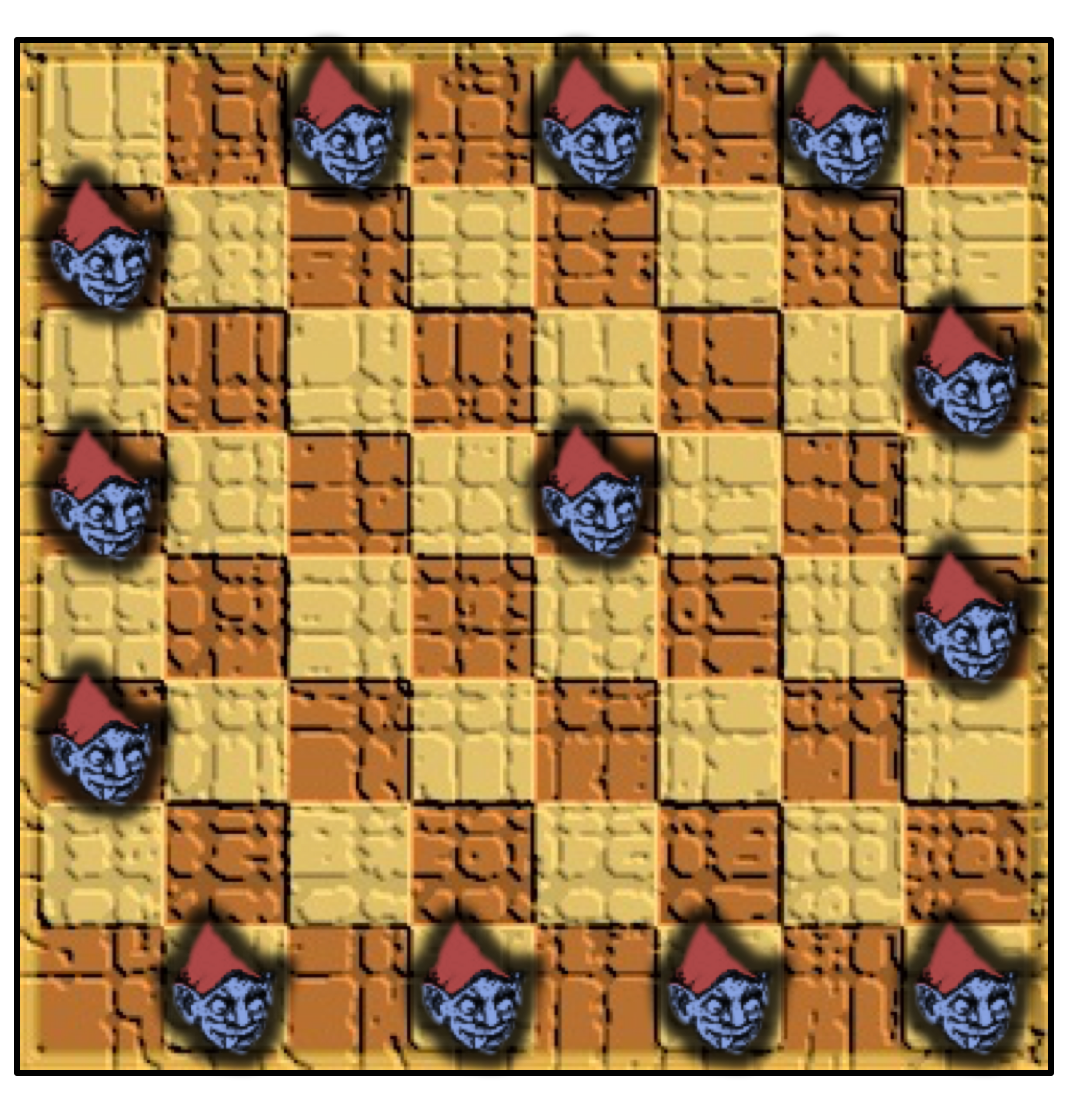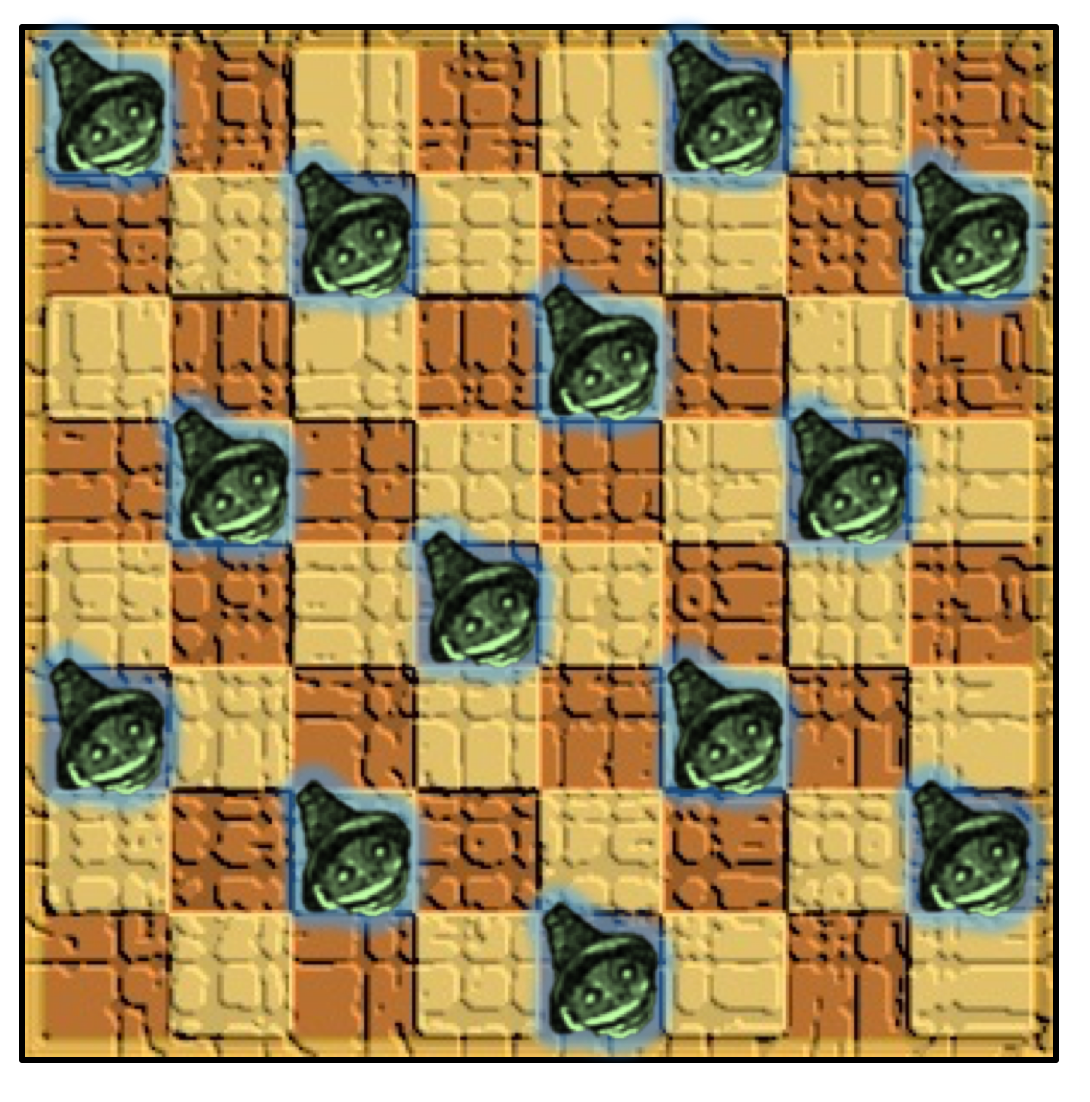# New Year Puzzle SolutionsThe solutions to the imp and pixie puzzles are below.

Solutions

The maximum number of imps that you can fit into the room is 13.

I find this solution particularly satisfying:There is also a solution in which two of the imps lie in the centre of the room (i.e. not against a wall). Can you find it? (It is unique, except for rotations and reflections.)

Proving that 13 is the maximum is fairly straightforward. Counting both colours of tile, the room has 15 diagonals running South-West to North-East and each can contain at most one imp. However, it is impossible to place imps on the first and second diagonals, since they would be adjacent, and it is impossible to place imps on the fourteenth and fifteenth diagonals for the same reason. This means that a maximum of 13 diagonals can be occupied, as they are in the solution given above.

The maximum number of pixies is also 13. Here is a possible solution:I won’t provide a complete proof of optimality, but here’s the basis of it (and there may well be a more efficient proof that I haven’t seen).

First observe that you can have no more than two pixies in a row. Next, consider all possible ways in which two pixies could be placed in a row. Counting rows starting with a dark tile and rows starting with a pale tile as distinct, there are 18 such possibilities. Clearly none of these rows can be included more than once in a possible solution.

Now observe that very few of these two-pixie rows can be adjacent. In fact, the only ones that can be (in either order and starting on either a pale or a dark tile) are:

```P _ _ _ _ P _ _ _ _ P _ _ _ _ P```

or:

```P _ _ _ _ _ _ P _ _ P _ _ P _ _ ```

Knowing this, we can also conclude that it is impossible to have three or more consecutive two-pixie rows. This means that the best solution we can hope for would involve 3 separate pairs of two-pixie rows and 2 one-pixie rows, in a 2, 2, 1, 2, 2, 1, 2, 2 pattern, for a total of 14 pixies.

However, since both the possible pairs of two-pixie rows identified above use the same four columns, including three such pairs would mean that these columns each contain three pixies, which is not permitted. This means that there can only be two pairs of adjacent two-pixie rows, so our previous best case scenario is impossible.

If there are only 2 pairs of two-pixie rows, then it is impossible to fit 6 two-pixie rows into the room, so our best possible solution would involve 5 two-pixie rows and 3 one-pixie rows, for a total of 13 pixies. Since that describes the solution given above, it must be optimal.